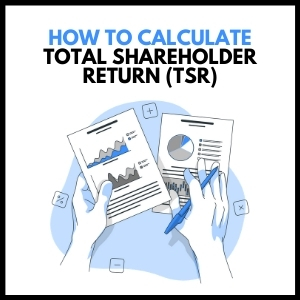# How to Calculate Total Shareholder Returns (TSR)In our earlier blog, Total Shareholder Return - Definition & Explanation we discussed about the meaning of Total Shareholder Returns and factors driving returns. In this blog, we will discuss how to calculate TSR if the investment period is more than one year.

## Example 1 – TSR calculation when there is no dividend payout.

John has purchased 1 share of ABC Limited for \$110 on 31st December 2015. ABC Limited has not paid any dividend till date. John wants to calculate the total returns earned annually byhim in ABC Limited from 31st December 2015 to 31st December 2020.

Here is a table showing the share price of ABC Limited for last 5 years from 31st December 2015 to 31st December 2020.TSR is total returns earned by an investor during the investment period. Investing in stock generate returns in 2 ways.

• Capital appreciation from share price increase
• Dividend yield

Go from beginner to an expert in Data Analytics Skill in Excel, Join the course and get discounts now!

Annual Capital appreciation is calculated as change in share price divided by share price at the beginning of the period.For example, Capital appreciation in 2016 = (115-110)/110 = 4.5%

Dividend yield is calculated as dividend earned during the period divided by the share price at the start of the year.In our example, there is no dividend, therefore the dividend yield is NIL.

Total shareholder return in this case, is just the share price appreciation.

The annual share price appreciation and dividend yield for all the years is shown in the below table. Total TSR in this example, is equal to the share price change.Here TSR calculation for the total investment period, can be done in two ways.

One is by calculating annualized share price appreciation, which is equal to the total TSR in this example.n = Total no of yearsIn the second method, we can compute the annualized return by using the Geomean function in excel.

To calculate Geomean, type =Geomean(1+(YoY return range))-1

Then press, Control + Shift + Enter (Since it is an array function). You get the same answer. TSR = 6%.

Learn more about our course on Valuation Analyst Skills Training (VAST) course to become an expert in business valuation.

## Example 2 – TSR calculation when there is dividend payout.

Let us look at another example. Here we have the share price and dividend payment by XYZ Limited for year ending 2015 to 2020. This Company has paid dividends annually.Total shareholder returns here is share price appreciation plus dividend yield.

Capital appreciation in 2016 = (115-110)/110 = 4.5%

Subscribe Now to avail an offer for a comprehensive course on statistical analysis

Dividend yield for 2016 = (Dividend paid/Starting period share price) = 6/110 = 5.5%

Total TSR for 2016 = 4.5% + 5.5% = 10%

The table below shows TSR calculation annually for each year.Suppose you had invested \$100 on 31st Dec 2015, your investment would have become \$110 on 31st Dec 2016 (ie, 100(1+10%))

In 2017, the investment would become \$92.3 (ie, 110*(1+(-16.1%))

We can calculate the value of investment annually (see table below).Investment of \$100 on 31st Dec 2015, would become \$186.3 on 31 st Dec 2020.We can also use the Geomean function in excel, to calculate annualized TSR.

To calculate Geomean, type =Geomean(1+(YoY returns))-1

= Geomean(1+(10%,-16.1%,44.4%,22.5%,14.1%))-1

Then press, Control + Shift + Enter (Since it is an array function).

You get the same answer. TSR = 13.3%.

Let us know your questions, by writing in the comments section.